Area
Exponents
Order of Operations
Random Riddles
Percent
100

This is the equation for finding the area of a rectangle.

A = lw

100

24

16

100

Simplify the following expression: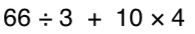10 x 4 = 40

66 / 3= 22

22 + 40 = 62

62

100

-4 + -5100

What has to be broken before you can use it?

an egg

100

What is 3/4 as a percent?

75%

200

This is the equation for finding the area of a triangle

A = (bh)/2 or 1/2bh

200

What is 93

729

200

Simplify the following expression: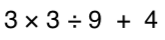3x3=9

9/9=1

1+4= 5

5

200

14 + -12

https://www.khanacademy.org/math/arithmetic/arith-review-negative-numbers/arith-review-add-negatives-intro/v/adding-negative-numbers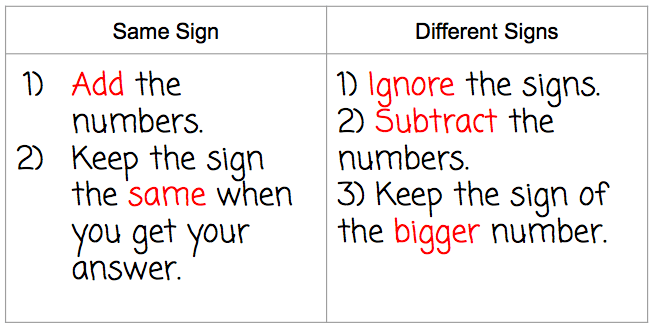200

What begins with T, finishes with T, and has T in it?

a teapot

200

What is 4/5 as a percent?

80%

300

This is the equation for finding the area of a circle300

what is 25

32

300

3 + 42 * (3 + 2)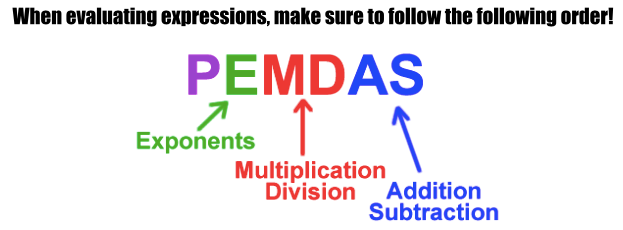300

-10 + 15300

What goes up but never comes back down?

300

95% is what as a fraction in simplest form?

19/20

400

This is how you find the area of a parallelogram.

A=bh

400

What is 5+ 58

390,750

400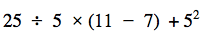400

-2 + -3 + 5

https://www.khanacademy.org/math/arithmetic/arith-review-negative-numbers/arith-review-add-negatives-intro/v/adding-negative-numbers400

No matter what, what can you always count on?

400

60% is what as a decimal?

0.60

500

If the radius of a circle is 7 inches, find the area of the circle.

49 PI sq.inches

500500

6 + (-2 + -5)

500

What is full of holes but still holds water?

a sponge

500

What is 1/3 as a percent?

33.3%

Click to zoom